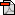GETSI Teaching Materials >Flood Hazards > Unit 2: Flood Frequency Analysis
GETSI's Earth-focused Modules for Undergraduate Classroom and Field CoursesLearn More
This module is part of a growing collection of classroom-tested materials developed by GETSI. The materials engage students in understanding the earth system as it intertwines with key societal issues. The collection is freely available and ready to be adapted by undergraduate educators across a range of courses including: general education or majors courses in Earth-focused disciplines such as geoscience or environmental science, social science, engineering, and other sciences, as well as courses for interdisciplinary programs.
Explore the Collection »Download

# Unit 2: Flood Frequency Analysis

## Summary

This unit introduces students to the statistical concepts that are used to determine the relationships between peak flow magnitude, return periods, and societal risk. The intent is that when a student hears or uses the term "100-year flood," they understand how it is obtained. The vocabulary and techniques of flood frequency analysis (FFA) are introduced through demonstrations. In a formative assessment exercise, students will use concepts learned in demonstrations to conduct an FFA in a new river.

Used this activity? Share your experiences and modifications

## Learning Goals

Students will be able to:

1. Define and compute exceedance probabilities and return periods for an annual peak flow time series
2. Apply probability distribution models to estimate flow magnitudes for return periods that are beyond the range of data
3. Explain relationships between probability and risk

## Context for Use

The content for Unit 2 is appropriate for upper division Geoscience and Engineering courses. Students should already understand basic statistical concepts, and should have completed Unit 1: Introduction to Flooding. Unit 2 can be considered optional in the module. However, later units use terms such as return period and exceedance probability that are explained in Unit 2. While students can understand what a return period is without completing this unit, here they will learn how it is computed and therefore gain a better understanding of it meaning.

## Description and Teaching Materials

Students learn through an online COMET tutorial, a presentation on flood frequency analysis (FFA), an in-class exercise in which the students conduct a simpler FFA FFA, and a longer FFA exercise, which can be finished up by the students as homework. The unit can be completed in one 50-minute class period with an additional preparation exercise and homework assignment.

Prior to class, students should complete the COMET tutorial Flood Frequency Analysis. Students will need to create a free account with MetEd.

In class, we recommend the instructor walking the students through the Middle Fork Boise River Excel file to explain some fairly complicated equations and calculations. This can be done in conjunction with the presentation in whatever way the instructor prefers. The students can start on the longer exercise using data from the Wabash River in class, if there is time, and complete the rest as homework.

## Teaching Notes and Tips

The student exercise is designed to demonstrate the basic concepts of flood frequency analysis. It is possible to simply assign the whole unit as a homework assignment. In this case, students would work through the presentation and homework assignment themselves. Alternatively, the instructor can work through the slides and Boise data file with the class. Students can complete the flood frequency analysis of the Wabash River by essentially recreating the Middle Fork Boise example in a new spreadsheet. Because the spreadsheet is "canned," students could complete the Wabash analysis by simply copying and pasting. Instructors should ensure that students actually work through the problem.

## Assessment

Formative assessments should happen, at the instructors discretion, to gauge understanding of key concepts identified in the instructors notes attached to each slide. Additionally, students should complete all quizzes contained in the Comet tutorial.

### Summative Assessment:

The summative assessment for this unit is the student exercise wherein students complete a flood frequency analysis of the Wabash River. Students duplicate the steps provided in the example analysis for the Middle Fork Boise River, and then answer discussion questions to ensure that they understand basic concepts. Some questions have definite right or wrong answers, given in the answer key. Where an open-ended answer is required, students can be assessed based on a simple 2-point system.

• 2 points = correct answer with thorough supporting evidence and/or complete description
• 1 point = answer not completely correct or lacking thorough supporting evidence or description
• 0 points = incorrect answer

## References and Resources

Already used some of these materials in a course?
Let us know and join the discussion »

Considering using these materials with your students?